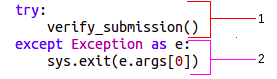Help Center

# Purpose

Every Data Science and Machine Learning question is conceptually different, therefore each question requires a unique evaluation metric. The evaluation metric in this case is a checker file. This file is used to evaluate a candidate's submission.

A checker file contains the following two functions:

1. gen_score: To generate the score after evaluation of the candidate’s submission.
2. verify_submission: To verify the file submitted by the candidate, you must perform some checks. Some of these checkpoints include checking the file type, number of rows present, number of columns present, predictions for all IDs provided in result.format, and so on.

# Programming languages supported

Checker file is supported in the following languages only:

1. Python 3
2. R

Note: HackerEarth uses Python 3 to create checker files. Thus, the screenshot of codes provided in the following sections in Python 3.

# Structure of a checker file

Importing libraries

You must import all the required libraries. Refer to the following image for a list of the required libraries.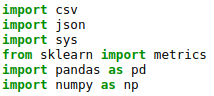The parts of this code and its description are as follows: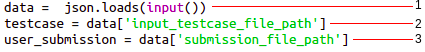1. A JSON file is loaded to the data variable. This file contains the path of the test case and user submission.
2. The testcase variable is assigned the path for the test case file.
3. The user_submission variable is assigned the path of the submission file.

## The gen_score() function

This function takes two arguments actual and predicted. The actual parameter represents the truth values of the target column(s) in the test case and the predicted parameter represents the predictions of the target column(s) in a candidate's submission file. Here, the score variable contains the formula based on the evaluation metric.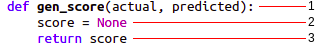Here, the descriptions of the labels is as follows:

1. The gen_score function with two parameters as mentioned.
2. Score is calculated based on an evaluation metric and is assigned to the score variable..
3. The function returns the value of score after the required calculation in the previous step.

Example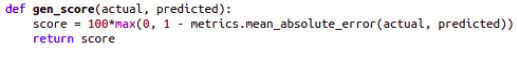## The verify_submission() function

1. The ID variable represents the name of the index or ID column. You are required to enter the name of the index or ID column in place of None.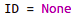Example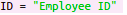2. The following line of code checks the type of the submission file submitted by the candidate such as whether it is in .csv format: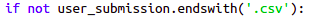If the file is not in .csv format, then the checker raises an exception and displays the following error message: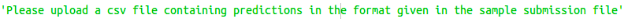3. The fp_submission variable (labelled as 1) reads the candidate's submission and the fp_testcase variable (labelled as 2) reads the test cases of the question.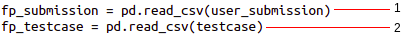4. The following line of code assigns the number of rows of the result file to the num_rows variable.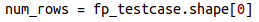The following line of code checks whether the number of rows of the submission file is equal to the number of rows of test case: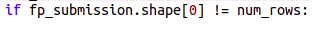If the numbers of rows are not equal, then the program raises an exception and displays the following error message: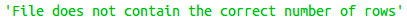5. The candidate's submission must contain the following:

• All the columns that are provided in the result file.
• All column names must be written in the same way as mentioned in the sample submission file.

To check this, if the set difference between the submission file’s columns and the result file’s columns must be equal to zero. This is performed by the following line of code: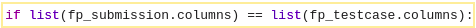In any case, if the submission file does not contain a column that is provided in the test case, then it raises an exception and the following error message is displayed: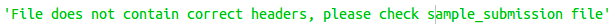6. The following two lines of code sets the index in the result file (labelled as 1) and candidate’s submission (labelled as 2):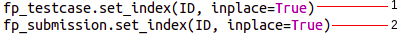7. The label_cols variable extracts the names (labels) of the columns that are available in the result file and candidate's submission.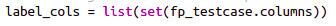8. You are required to determine all unique IDs that are available in the result file and candidate's submission. All the common index values or unique IDs are stored in this variable.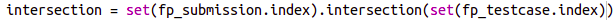9. Sometimes, a candidate can miss certain index values or unique IDs. You are required to check the unique IDs that are available in test cases but are not available in the candidate's submission. This is performed by using the following code of line: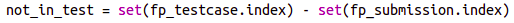Here, the not_in_test variable stores the indexes that are missing in the candidate’s submission file.
Further, if the length of not_in_test is equal to 0, that is, there are no missing index values, then the submission is accepted.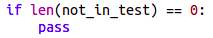If there are missing index values, then these values are stored in the following key_not_found variable.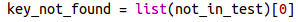All the missing index values are displayed in the following error message: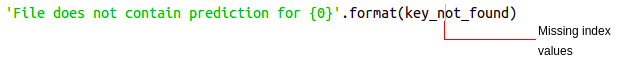10. The following two lines of code allows you to select the predictions across the index values that are common between the result file (labelled as 1) and candidate’s submission file (labelled as 2).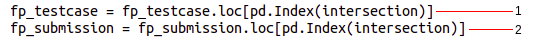11. The required predictions for all the unique IDs in the result file and the candidate's submission are initialized in the actual_values (labelled as 1) and predicted_values (labelled as 2) variables, respectively.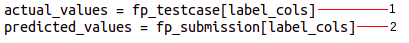Then, the actual values and predicted values are passed in the gen_score function. This is shown in the following line of code: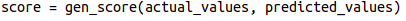12. The gen_score returns the score and it is printed up to six decimal places.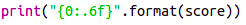13. The program begins with the verify_submission function (labelled as 1). If an exception is raised based on the checkpoints provided in the verify_submission function, it is displayed to the candidate (labelled as 2). This is performed by using the following lines of code: